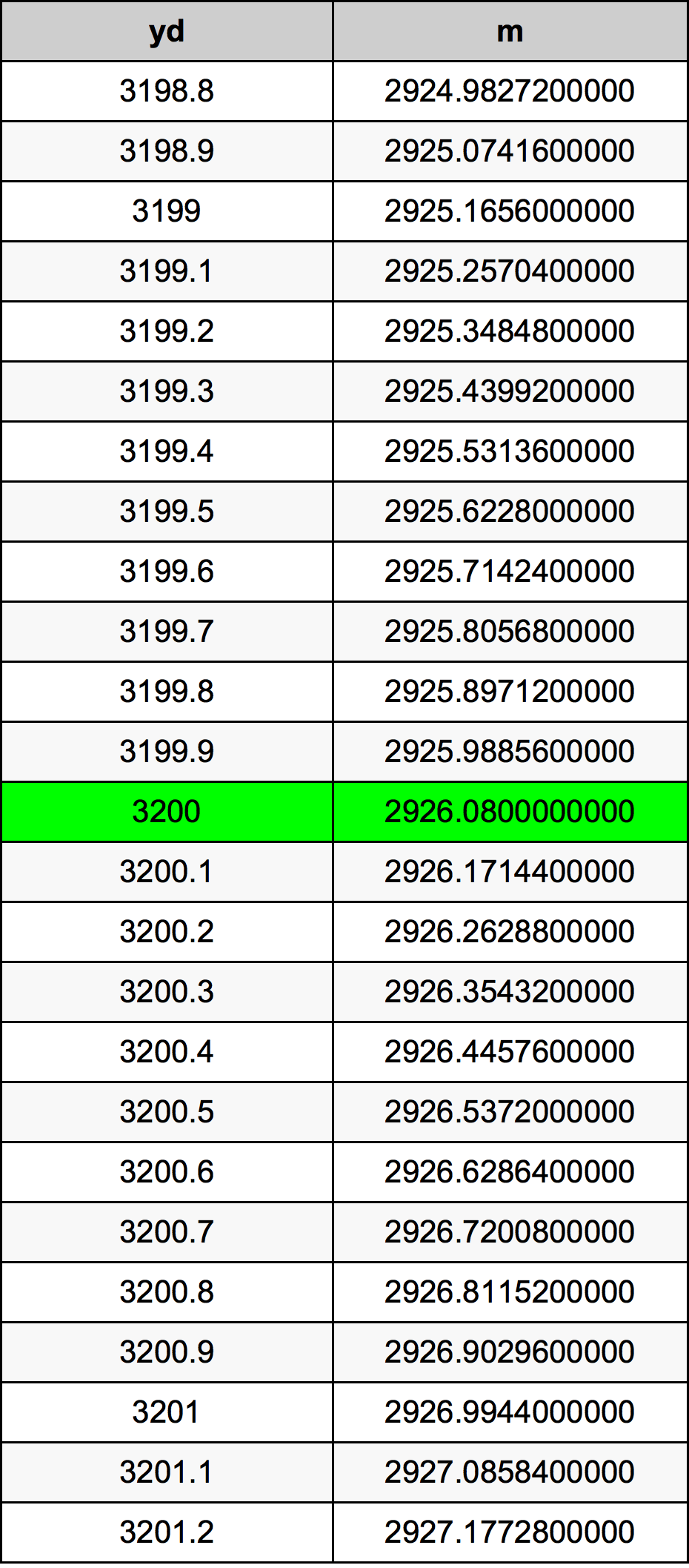Yards To Meters

# 3200 yd to m3200 Yards to Meters

yd
=
m

## How to convert 3200 yards to meters?

 3200 yd * 0.9144 m = 2926.08 m 1 yd
A common question is How many yard in 3200 meter? And the answer is 3499.56255468 yd in 3200 m. Likewise the question how many meter in 3200 yard has the answer of 2926.08 m in 3200 yd.

## How much are 3200 yards in meters?

3200 yards equal 2926.08 meters (3200yd = 2926.08m). Converting 3200 yd to m is easy. Simply use our calculator above, or apply the formula to change the length 3200 yd to m.

## Convert 3200 yd to common lengths

UnitLength
Nanometer2.92608e+12 nm
Micrometer2926080000.0 µm
Millimeter2926080.0 mm
Centimeter292608.0 cm
Inch115200.0 in
Foot9600.0 ft
Yard3200.0 yd
Meter2926.08 m
Kilometer2.92608 km
Mile1.8181818182 mi
Nautical mile1.5799568035 nmi

## What is 3200 yards in m?

To convert 3200 yd to m multiply the length in yards by 0.9144. The 3200 yd in m formula is [m] = 3200 * 0.9144. Thus, for 3200 yards in meter we get 2926.08 m.

## 3200 Yard Conversion Table## Alternative spelling

3200 Yards to Meter, 3200 Yards in Meter, 3200 Yards to Meters, 3200 Yards in Meters, 3200 Yard to Meter, 3200 Yard in Meter, 3200 Yard to Meters, 3200 Yard in Meters, 3200 Yard to m, 3200 Yard in m, 3200 yd to Meter, 3200 yd in Meter, 3200 Yards to m, 3200 Yards in m